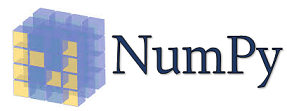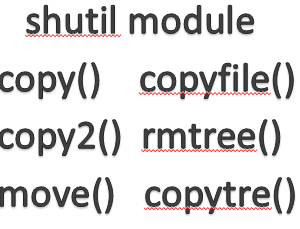## Numpy Array ManipulationNumpy is an acronym for “Numeric Python” or “Numerical Python“.It is an open source core library for scientific computing(mathematical, logical) in Python.NumPy supports a large number of mathematical operations.

In Numpy Several routines are available for manipulation of elements in ndarray object. Some of they are classified as below

## .Changing Shape

### flat

This function is used to returns a 1-D iterator over the array.
This function behaves similar to Python’s built-in iterator.

Note
This function is not available for List of ndarrays
It does not depend on the order i.e C and F
C(read/write the elements using C-like index order)
F(read/write the elements using Fortran-like index order)

Syntax

```numpy.ndarray.flat
numpy.ndarray.flat[i]
where i is index number
```

Example

```>>> import numpy as np
>>> a1=np.array([9,8,6,3,2,1,5,4,7])
>>> a=a1.reshape(3,3,order='F')
>>> a  #The Original array
array([[9, 3, 5],
[8, 2, 4],
[6, 1, 7]])

>>> a.flat
>>> 8  #After applying thflat function

>>> a1=np.array([9,8,6,3,2,1,5,4,7])
>>> a=a1.reshape(3,3,order='C')
>>> a  #The Original array
array([[9, 8, 6],
[3, 2, 1],
[5, 4, 7]])

>>> a.flat
>>> 3  #After applying the flat function
```

An assignment example using a flat function

```>>> a1=np.array([9,8,6,3,2,1,5,4,7])
>>> a=a1.reshape(3,3,order='F')
>>> a  #The Original array
array([[9, 3, 5],
[8, 2, 4],
[6, 1, 7]])

>>> a.flat=9
>>> a.flat[[1,3]]=1  #We can use more than one index number

>>> a  #After applying the flat function
array([[9, 1, 5],
[1, 2, 9],
[6, 1, 7]])
```

### flatten

Flatten returns a copy of single dimensional array by merging all sub-arrays.
This is a function of ndarray object
Note-This function is not available for List of ndarrays object

Syntax

```numpy.ndarray.flatten(order='C')

Parameter
order(optional):'C','F','A','K'
Default order is C
'C' means to flatten in row-major(C-style) order.
'F' means to flatten in column-major (Fortran-style) order.
'A' means to flatten in column-major order if a is Fortran contiguous in memory, row-major order otherwise.
'K' means to flatten a in the order the elements occur in memory.

```

Example

```>>> import numpy as np

>>> a = np.array([[1,5], [3,4]])
>>> a.flatten(order='C')
>>> a.flatten() #Default order is C
array([1, 5, 3, 4])

>>> a.flatten(order='F')
array([1, 3, 5, 4])```

### ravel

Ravel is a library level function i.e numpy.
This function returns a contiguous flattened array.
Return array will have the same type as the input array.
Syntax

```numpy.ravel(arr,order='C')

Parameter

arr-input array
order-optional-{'C','F','A','K'}
Default order is C
'C' means to flatten in row-major(C-style) order.
'F' means to flatten in column-major (Fortran-style) order.
'A' means to flatten in column-major order if a is Fortran contiguous in memory, row-major order otherwise.
'K' means to flatten a in the order the elements occur in memory.
```

Example

```>>> import numpy as np

>>> a = np.array([(1,2,3,4),(3,1,4,2)])   #Shape of array a is (2, 4)
>>> b = a.ravel()   #np.ravel(a)

>>> b   #Shape of array b is (8,)
array([1, 2, 3, 4, 3, 1, 4, 2])

It is equivalent to reshape(-1, order=order).

>>> b= a.reshape(-1)
>>> b
array([1, 2, 3, 4, 3, 1, 4, 2])

>>>  b=a.ravel(order='F')   #np.ravel(a,order='F')
>>> b
array([1, 3, 2, 1, 3, 4, 4, 2])
```

When an order is ‘A’, it will preserve the array‘s ‘C‘ or ‘F‘ ordering:

```>>> b=a.ravel(order='A')
>>> b
array([1, 2, 3, 4, 3, 1, 4, 2])
```

When an order is ‘K’, it will preserve orderings that are neither ‘C’ nor ‘F’, but won’t reverse axes:

```>>> a = np.arange(12).reshape(2,3,2).swapaxes(1,2)
>>> a
array([[[ 0,  2,  4],
[ 1,  3,  5]],
[[ 6,  8, 10],
[ 7,  9, 11]]])

>>> a.ravel(order='C')
array([ 0,  2,  4,  1,  3,  5,  6,  8, 10,  7,  9, 11])

>>> a.ravel(order='K')
array([ 0,  1,  2,  3,  4,  5,  6,  7,  8,  9, 10, 11])
```

### reshape

This function gives a new shape to an array without changing the data.
It is not always possible to change the shape of arrays without copying the data.

Syntax

```numpy.ravel(arr,newshape,order)

arr-Array to be reshaped.
newshape : The new shape should be compatible with the original shape.
order : {'C', 'F', 'A'}, optional
'C' means to read / write the elements using C-like index order
'F' means to read / write the elements using Fortran-like index order,
'A' means to read / write the elements in Fortran-like index order if a is Fortran contiguous in memory, C-like order otherwise.
```

Example

```>>> a =np.array([[10,20,30], [40,50,60]])
>>> np.reshape(a,6,order='C') # same as np.reshape(a,6)
array([10, 20, 30, 40, 50, 60])

>>> np.reshape(a,6,order='F')
array([10, 40, 20, 50, 30, 60])

>>>np.reshape(a,(3,2))
array([[10, 20],
[30, 40],
[50, 60]])
```

Category:Hi, I’m Steven, a Florida native, who left my career in corporate wealth management six years ago to embark on a summer of soul searching that would change the course of my life forever.

• ## The term ‘npm’ is not recognized as the name of a cmdlet, function, script file, or operable program• ## Exploring Essential File Operations in Python with shutil library